# Test: Relational Algebra, Tuple Calculus & SQL- 1

## 10 Questions MCQ Test GATE Computer Science Engineering(CSE) 2023 Mock Test Series | Test: Relational Algebra, Tuple Calculus & SQL- 1

Description
Attempt Test: Relational Algebra, Tuple Calculus & SQL- 1 | 10 questions in 30 minutes | Mock test for Computer Science Engineering (CSE) preparation | Free important questions MCQ to study GATE Computer Science Engineering(CSE) 2023 Mock Test Series for Computer Science Engineering (CSE) Exam | Download free PDF with solutions
QUESTION: 1

### The relational algebra expression equivalent to the following expression: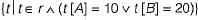is:

Solution:

The expression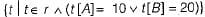can be represented in relational algebra as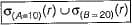It says that select those rows from relation r where A = 10 union where B = 20.
Hence option (b) is correct.

QUESTION: 2

Solution:
QUESTION: 3

### Which of the following statements is correct with respect to entity integrity?

Solution:

According to entity integrity primary key of relation should not contain null values.

QUESTION: 4

Which of the following operations is not part of the five basic set operations in relational algebra?

Solution:

Five primitive operators of Codd’s algebra are the selection, the projection the Cartesian product, the set union and the set difference.

QUESTION: 5

Which of the following relational algebraic operation is not a commutative operation?

Solution:
QUESTION: 6

Consider a banking database with the following relation with three attributes loans (br_name, loan_no, amount).
Find the appropriate query for the given statements below:
“Find the loan number for each loan of an amount greater than 20000”

Solution:

Loans (br_name, loan_no, amount)
For the query “find the loan number for each loan of an amount greater than 20000”.
We have to check for each loan to find a loan number which is greater than 20,000.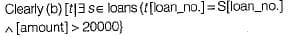Hence (b) is the correct option.

QUESTION: 7

Let R(a, b, c) and S(d, e, f) be two relations in which d is the foreign key of S that refers to the primary key of R. Consider the following four operations R and S
1. Insert into R
2. Insert into S
3. Delete from R
4. Delete from S
Which of the following is true about the referential integrity constraint above?

Solution:

Referential integrity constraint: In relational model, two relation are related to each other over the basis of attributes, Every value of referencing attribute must be null or be available in the referenced attribute.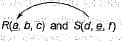Here d is the foreign key of S that refers to the primary key of R.
1. Insert into R will not cause any violation.
2. Insert into S may cause violation because for each entry in ‘S ’ it must be. in ‘R ’ .
3. Delete from R may cause violation because for the deleted entry in R there may be referenced entry in the reIation S.
4. Delete from S will not cause any violation.
Hence (d) is the correct option.

QUESTION: 8

Let r and s be two relations over the relation schemes R and S respectively, and let A be an attribute in R. Then the relational algebra expression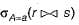is always equal to

Solution:

The relational algebra expression: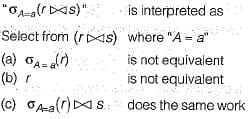Hence (c) is the required option.

QUESTION: 9

Join Selectivity of a relation R in a natural join with a relation S is the _____

Solution:
QUESTION: 10

Which of the following is wrong?

Solution: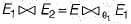is not equivalent because θ join is not cumulative hence the entries in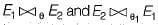may not be same. Hence option (d) is correct.Use Code STAYHOME200 and get INR 200 additional OFF Use Coupon Code